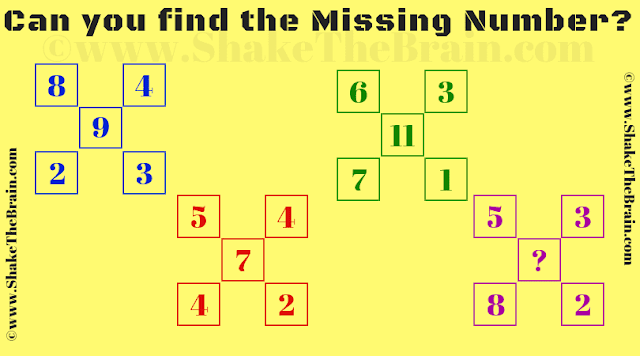In this Maths puzzle find the Mathematical pattern with which these given numbers are related and then find the missing number which will replace the question mark. Solving this puzzle will help you to improve your IQ. Let us see if you can find the missing number?Can you find the value of the missing number?
The answer to this "Maths Puzzle", can be viewed by clicking on the answer button.